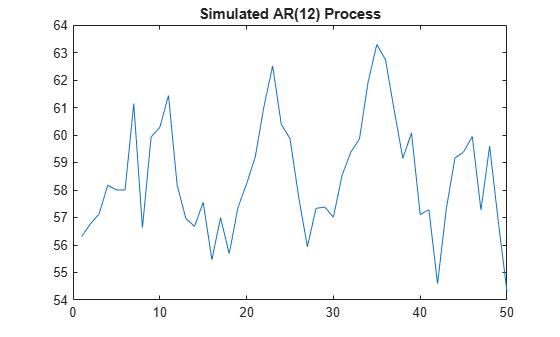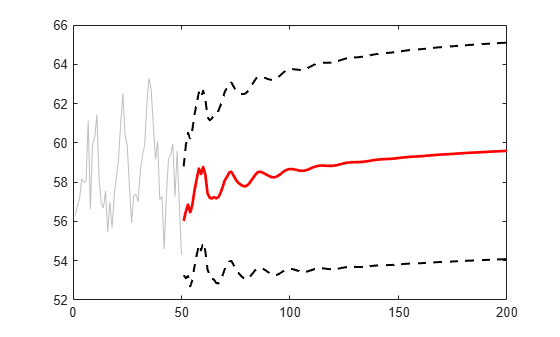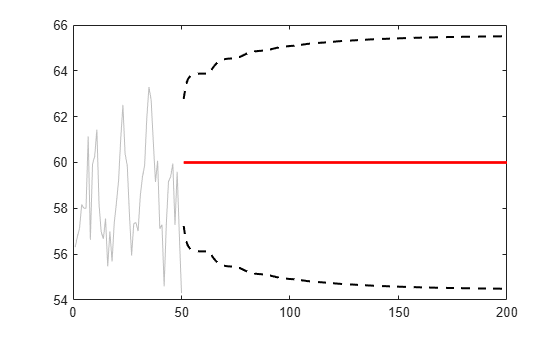Documentation

## Convergence of AR Forecasts

This example shows how to forecast a stationary AR(12) process using `forecast`. Evaluate the asymptotic convergence of the forecasts, and compare forecasts made with and without using presample data.

### Step 1. Specify an AR(12) model.

Specify the model

`${y}_{t}=3+0.7{y}_{t-1}+0.25{y}_{t-12}+{\epsilon }_{t},$`

where the innovations are Gaussian with variance 2. Generate a realization of length 300 from the process. Discard the first 250 observations as burn-in.

```Mdl = arima('Constant',3,'AR',{0.7,0.25},'ARLags',[1,12],... 'Variance',2); rng('default') Y = simulate(Mdl,300); Y = Y(251:300); figure plot(Y) xlim([0,50]) title('Simulated AR(12) Process')```### Step 2. Forecast the process using presample data.

Generate forecasts (and forecast errors) for a 150-step time horizon. Use the simulated series as presample data.

```[Yf,YMSE] = forecast(Mdl,150,Y); upper = Yf + 1.96*sqrt(YMSE); lower = Yf - 1.96*sqrt(YMSE); figure plot(Y,'Color',[.75,.75,.75]) hold on plot(51:200,Yf,'r','LineWidth',2) plot(51:200,[upper,lower],'k--','LineWidth',1.5) xlim([0,200]) hold off```The MMSE forecast sinusoidally decays, and begins converging to the unconditional mean, given by

`$\mu =\frac{c}{\left(1-{\varphi }_{1}-{\varphi }_{12}\right)}=\frac{3}{\left(1-0.7-0.25\right)}=60.$`

### Step 3. Calculate the asymptotic variance.

The MSE of the process converges to the unconditional variance of the process (${\sigma }_{\epsilon }^{2}=2$). You can calculate the variance using the impulse response function. The impulse response function is based on the infinite-degree MA representation of the AR(2) process.

The last few values of `YMSE` show the convergence toward the unconditional variance.

```ARpol = LagOp({1,-.7,-.25},'Lags',[0,1,12]); IRF = cell2mat(toCellArray(1/ARpol)); sig2e = 2; variance = sum(IRF.^2)*sig2e % Display the variance```
```variance = 7.9938 ```
`YMSE(145:end) % Display the forecast MSEs`
```ans = 6×1 7.8870 7.8899 7.8926 7.8954 7.8980 7.9006 ```

Convergence is not reached within 150 steps, but the forecast MSE is approaching the theoretical unconditional variance.

### Step 4. Forecast without using presample data.

Repeat the forecasting without using any presample data.

```[Yf2,YMSE2] = forecast(Mdl,150); upper2 = Yf2 + 1.96*sqrt(YMSE2); lower2 = Yf2 - 1.96*sqrt(YMSE2); YMSE2(145:end) % Display the forecast MSEs```
```ans = 6×1 7.8870 7.8899 7.8926 7.8954 7.8980 7.9006 ```
```figure plot(Y,'Color',[.75,.75,.75]) hold on plot(51:200,Yf2,'r','LineWidth',2) plot(51:200,[upper2,lower2],'k--','LineWidth',1.5) xlim([0,200]) hold off```The convergence of the forecast MSE is the same without using presample data. However, all MMSE forecasts are the unconditional mean. This is because `forecast` initializes the AR model with the unconditional mean when you do not provide presample data.